• 方便神经网络学习使用的神经网络算法matlab程序，用于初步学习
• BP神经网络是一个前向网络，它利用误差反向传播算法对网络进行训练，结构简单，可塑性强。本例选择3层BP神经网络（隐层为1层）来逼近函数，单输入单输出，隐层包含7个神经元，预设精度为0.1，学习率设为0.1，循环...
• 模拟退火禁忌搜索遗传算法神经网络MATLAB程序合集-模拟退火，禁忌搜索，遗传算法神经网络-MATLAB程序合集.rar 模拟退火，禁忌搜索，遗传算法神经网络-MATLAB程序合集.rar
• matlab神经网络算法源码。 使用matlab BP神经网络算法区分两种类型。 内含源码和测量的数据
• 模拟退火，禁忌搜索，遗传算法神经网络MATLAB程序实现，里面集合了这几种算法的matlab实现代码，有感兴趣学习的朋友可以看一看
• 模拟退火，禁忌搜索，遗传算法神经网络-MATLAB程序合集.zip
• 简单神经网络算法Matlab程序，附带教程，Matlab2014可直接运行。 1、代码里的函数已修改为"Matlab2014a"版本，具体为mapminmax和newff两个函数。 2、保存的网页为改代码对应的讲义，十分简洁易懂。 3、资源仅供...
• 模拟退火，禁忌搜索，遗传算法神经网络-MATLAB程序合集，经典的常用Maltb算法
• Matlab遗传算法优化神经网络权值的程序-遗传算法优化神经网络权值的程序.rar 遗传算法优化神经网络权值的程序
• 自己搜集的神经网络算法，包含MATLAB程序，可以用于数学建模学习与机器学习，比较全面
• 将IMPSO算法训练的BP神经网络分别应用于齿轮热处理中硬化层深的预测以及用于柴油机的缸盖与缸壁的故障诊断中，并将预测结果、诊断结果与BP神经网络、标准粒子群优化算法训练的BP神经网络的实验结果进行对比，实验...
• matlab神经网络程序算法，对做优化控制的有用
• matlab神经网络工具箱使用最近看了台湾大学林轩田老师的公开课视频，作为机器学习的入门者，记录下来以供查阅。问题模型 threshold表示界限值。 sign(x)根据x大于0和小于0分别取+1和-1。 将-threshold作为w0 也...

问题模型
PLA算法
matlab程序
matlab神经网络工具箱使用

最近看了台湾大学林轩田老师的公开课视频，作为机器学习的入门者，记录下来以供查阅。

问题模型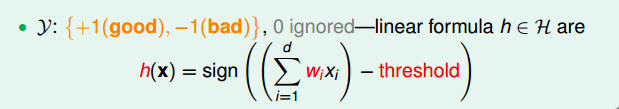threshold表示界限值。
sign(x)根据x大于0和小于0分别取+1和-1。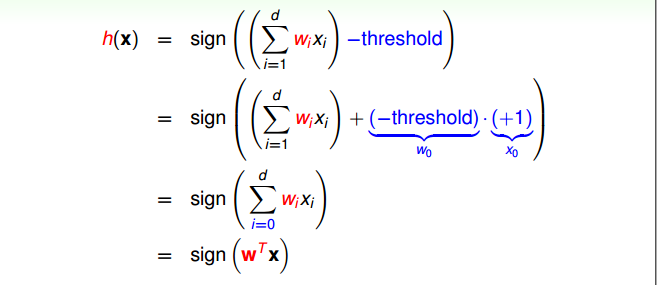将-threshold作为w0 也就是作为w⃗ <!--//--><![CDATA[//><!--
\vec{w}
//--><!]]>的第一个分量。

因此我们需要求出w⃗ <!--//--><![CDATA[//><!--
\vec{w}
//--><!]]>通过给定的训练值x⃗ <!--//--><![CDATA[//><!--
\vec{x}
//--><!]]>

PLA算法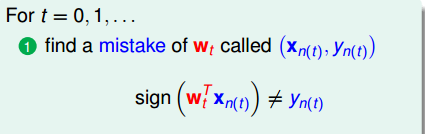错误分为两种。

wTtxn(t)>0<!--//--><![CDATA[//><!--
w_t^Tx_{n(t)} > 0
//--><!]]> 但 yn(t)=−1<!--//--><![CDATA[//><!--
y_{n(t)} = -1
//--><!]]> ，如下图, 更新wt+1=wt−x<!--//--><![CDATA[//><!--
w_{t+1}=w_t - x
//--><!]]>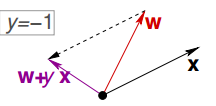wTtxn(t)<0<!--//--><![CDATA[//><!--
w_t^Tx_{n(t)} < 0
//--><!]]> 但 yn(t)=1<!--//--><![CDATA[//><!--
y_{n(t)} = 1
//--><!]]> ，如下图，更新wt+1=wt+x<!--//--><![CDATA[//><!--
w_{t+1}=w_t + x
//--><!]]>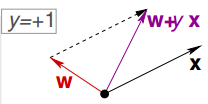总结情况如下图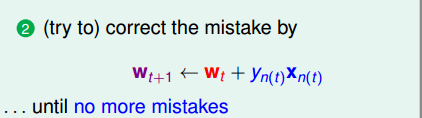以上算法在数据线性可分时必收敛。

matlab程序

先随机数据ϵ[0,10]<!--//--><![CDATA[//><!--
\epsilon[0, 10]
//--><!]]> ，随机y为{1, -1}控制点为圆圈或叉。

x0 = ones(20, 1);
x1 = rand(20, 2)*10;
x = [x0, x1];
test = rand(20, 1);
y = ones(20, 1);
for i=1:20
if x1(i, 1)>6
y(i) = -1;
end
end

j=1;
for i=1:20
if y(i)==-1
u(j) = x1(i, 1);
v(j) = x1(i, 2);
j = j+1;
end
end
scatter(u, v, 'or');
hold on;

j=1;
u=[];
v=[];
for i=1:20
if y(i)==1
u(j) = x1(i, 1);
v(j) = x1(i, 2);
j = j+1;
end
end
scatter(u, v, 'xk');
hold on;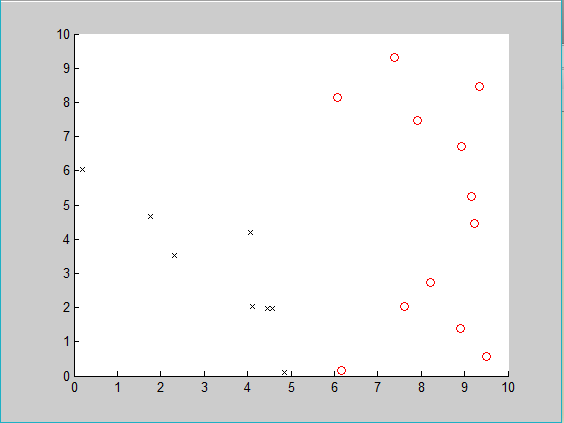通过改错更新w<!--//--><![CDATA[//><!--
w
//--><!]]>

w = [0, 0, 0];
while true
pd = false;
for i=1:20
t = x(i, :);
if w*t'*y(i)<=0
w = w + y(i)*t;
pd = true;
break;
end

end

if pd == false
break;
end
end
w
v = linspace(0, 10, 100);
u = -w(3)/w(2)*v - w(1)/w(2);
plot(u, v, '.');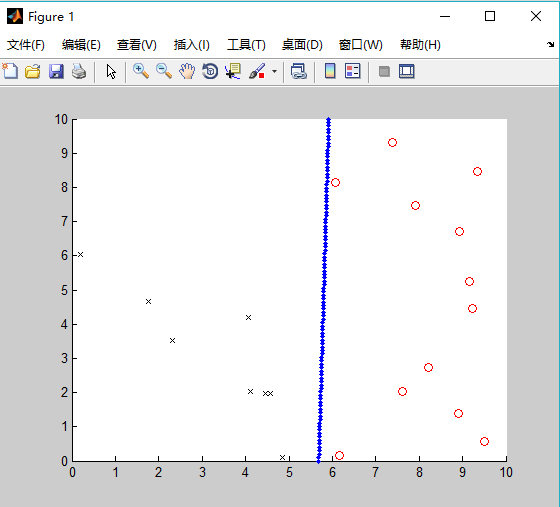matlab神经网络工具箱使用

以上的算法也可通过神经网络工具箱中单层感知元建立来完成。

net = newp(P, T);//创建一个感知器
其中P是一个R*2矩阵，其中R为感知器网络中输入向量的维数。
矩阵的每一行代表输入向量没个分量取值范围。
T是输出节点个数。

net = train(net, P, T);//训练感知器网络
P是R*Q矩阵，每一列是一个输入向量。
T是网络期望输出，S*Q矩阵，每一列是一个输出向量。

具体程序如下。

t = 1;
y(y==-1)=0;
net = newp([0, 10; 0, 10], t);
net = train(net, x1', y');

newt = sim(net, x1');
iw = net.iw;
b = net.b;
ww = [b{1}, iw{1}];
vv = linspace(0, 10, 100);
uu = -ww(3)/ww(2)*v - ww(1)/ww(2);
plot(uu, vv, '.k');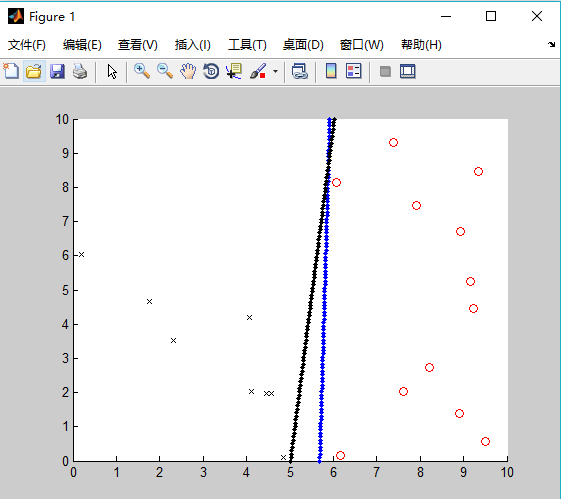黑色的线为第二次画的线，可以看出这两条线都将圆圈和叉分开了
展开全文人工智能
• 网上找的BP神经网络算法程序和大家分享分享-用matlab编BP神经网络预测程序.doc 内容在上传附件里面，有一点乱，但是还是可以借鉴参考，希望能有所帮助！我不太懂，大家可以相互探讨！
• 模拟退火，禁忌搜索，遗传算法神经网络-MATLAB程序合集
• 程序直接能够运行，当然对于matlab的版本可能有些要求，我的是2012版本没问题。这里边包括小波分析、神经网络以及遗传算法，实现对数据充分的拟合，直接输出误差变化过程图，可清楚的观察误差的过程。
• 首先，数学建模比赛一般分为优化类型的题目和数据分析或评价类的题目，需要3-4天提交一篇论文，三个成员需要有一名写手、一名编程人员和一名统筹调度（建模和想...编程人员需要熟练掌握Matlab、SPSS、Lingo，都很简单。
• Matlab遗传算法优化BP神经网络程序-遗传算法优化BP神经网络程序.rar 遗传算法优化BP神经网络程序
• 利用GA-BP神经网络算法的应用实例之MATLAB程序，有很详细的中文注释，大家可以根据实际的数据修改相应的参数！
• 使用bp神经网络算法实现异或问题的权值优化，程序模型为：输入层两个节点，中间层两个节点，输出层一个节点。各层节点数可调。
• 神经网络bp算法程序，三层神经网络，实现异或算法
• matlab 编写的神经网络bp算法程序。计算机仿真课的结课作业。上传共享。有L-M和贝叶斯两种优化算法
• 利用遗传算法优化BP神经网络，达到很好的权值和阈值
• Matlab的BP神经网络各种不同算法程序Matlab的BP神经网络各种不同算法程序Matlab的BP神经网络各种不同算法程序
• 基于遗传算法的BP神经网络优化算法matlab程序，matlab可以直接运行
• 这里边包括小波分析、神经网络以及遗传算法，实现对数据充分的拟合，直接输出误差变化过程图，可清楚的观察误差的过程。另外，对于学习小波神经网络以及搞中长期预报的同学可谓是在珍贵不过的程序了。
• BP神经网络整定的PID算法_matlab程序神经网络的PID算法MATLAB程序代码...

# 神经网络算法matlab程序matlab 订阅